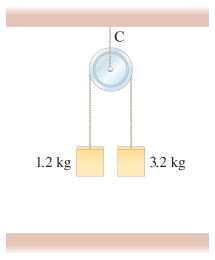# Problem: Suppose the pulley in the figure is suspended by a cord C.Determine the tension in this cord after the masses are released and before one hits the ground. Ignore the mass of the pulley and cords.

###### FREE Expert Solution

In this problem, we're asked to determine the tension in a system involving multiple objects.

You might remember this kind of arrangement is called an Atwood Machine.

As usual for any problem that involves a system of objects, we'll follow these steps:

1. Draw free-body diagrams for each object we're interested in.
2. Write Newton's Second Law Equation ∑F=ma for each object, remembering that is the same for both
3. Solve for the target variable

In step 1, we'll need a positive direction of motion to guide the sign conventions for the motion of the two masses.

99% (474 ratings)###### Problem Details

Suppose the pulley in the figure is suspended by a cord C.

Determine the tension in this cord after the masses are released and before one hits the ground. Ignore the mass of the pulley and cords.